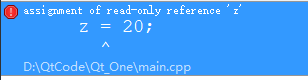## C++11新特性之auto关键字的使用 转

o
osc_wws45aot

auto这个关键字并不是一个全新的关键字，在旧标准中，它代表的是“具有自动存储期的局部变量”；但是它在这方面并没有起到很大的作用，比如：auto int i = 10 与int i = 10是等价的， 在旧标准中我们很少会用到auto关键字，因为非静态变量在默认的情况下本就是“具有自动存储期的”。

``````#include <iostream>

using namespace std;

int main()
{
int x = 10;
auto *a = &x;       //auto被推导为int
auto b = &x;        //auto被推导为int*
auto &c = x;        //auto被推导为int
auto d = c;         //auto被推导为int

const auto e = x;   //auto被推导为int
auto f = e;         //auto被推导为int

const auto& g = x;  //auto被推导为int
auto& h = g;        //auto被推导为int

return 0;
}``````

A.d的推导结果说明了当表达式是一个引用类型时，auto会把引用类型抛弃，直接推导成原始类型int;

B.f的推导结果说明了当表达式带有const属性时，auto会把const属性抛弃掉，直接推导成int类型;

C.g和h的推导说明了当auto和引用(换成指针在这里也将得到同样的结果)结合时，auto的推导将保留变量的const属性

``````#include <iostream>

using namespace std;

int main()
{
int x = 10;
const auto z = x;
auto y = z;
y = 10;
cout << y << endl;

const int a = 10;
a = 20;
cout << a << endl;

return 0;
}``````a现在只是一个可读变量，不可在更改它的初始值；所以说，如果上述y变量类型推导为const int，再为y赋值的话，在编译时会报出同样的错误，但在编译时并未出现上述错误，那就说明在进行类型推导时，把const属性给抛弃掉了，只保留了一个原始类型int.

``````#include <iostream>

using namespace std;

int main()
{
int x = 10;
const auto& z = x;
z = 20;
cout << z << endl;

return 0;
}``````(1).当不声明为指针或引用时，auto的推导结果和初始化表达式抛弃引用和cv限定符(const和volatile限定符的统称)。

(2).当声明为指针或引用时，auto的推导结果将保持初始化表达式的cv属性。

auto关键字不是说在任何地方都能适用的；

(1).不能作为函数的形参

(2).不能用于非静态成员变量

(3).auto无法定义数组

(4).auto无法推导出模板参数

``````#include <iostream>
#include <list>
using namespace std;

struct MyStruct
{
auto nValue = 0;  //错误： 非静态的成员变量
static const auto value = 10;
};

void MyPrintf(auto i) //警告：在参数声明中使用“auto”只能用-STD= C++1Y或-STD= GNU+1Y：
{
cout << i << endl;
}

int main()
{
auto array = {0};   //错误：无法定义数组
list<auto> MyList;      //错误：无法推导出模板的参数类型
auto i = 10;
MyPrintf(i);
return 0;
}``````

``````#include <iostream>
#include <vector>

using namespace std;

int main()
{
vector<int> MyVector;
//插入数据
for(int i = 0; i < 10; i++)
{
MyVector.push_back(i);
}
//旧特性中遍历数据
vector<int>::iterator it = MyVector.begin();
for(; it != MyVector.end(); it++)
{
cout << *it <<",";
}
cout << endl;
//新特性中遍历数据
auto MyIt = MyVector.begin();
for(; MyIt != MyVector.end(); MyIt++)
{
cout << *MyIt << ",";
}
return 0;
}``````

o

### osc_wws45aot#### 暂无文章

Mysql主从同步

1主从同步 1.1Master 1.1.1配置--编辑 my.cnf #编辑 mysql 的 /etc/my.cnf 配置文件vi /etc/my.cnf#添加如下配置server-id=1 #设置服务 IDlog_bin=mysql-bin #启动 binlog...

14分钟前
0
0

tankb52
28分钟前
13
0
2020最新微信域名防封技术 微信域名防封系统是如何操作的

51分钟前
11
0
mysql 为什么 SQL 语句不要过多的 join？

edison_kwok
52分钟前
17
0

4
0